# What do the Machine Learning Training and Validation graphs tell us?

If you’re like me and you think the Training and Validation graph looked good because it was smooth, or heading in a certain direction, but wasn’t really sure why, then you’re in the right place.

I know it can get confusing, but every graph is telling a story. And I like stories. Especially ones with happy endings.

In my last article, A Simple Maths Free PyTorch Model Framework, I proposed a simple framework to build models for Classification (Binary, Multi Class or Multi Label) and Regression, but more importantly a Maths Free approach of understanding what it was doing, and why.

Let’s start off with some simple boilerplate code to set up a reusable model framework.

`from sklearn.model_selection import train_test_splitfrom sklearn.datasets import  make_classificationimport torchfrom torch.utils.data import Dataset, DataLoaderimport torch.optim as torch_optimimport torch.nn as nnimport torch.nn.functional as Fimport numpy as npimport matplotlib.pyplot as pltclass MyCustomDataset(Dataset):    def __init__(self, X, Y, scale=False):        self.X = torch.from_numpy(X.astype(np.float32))        self.y = torch.from_numpy(Y.astype(np.int64))        def __len__(self):        return len(self.y)        def __getitem__(self, idx):        return self.X[idx], self.y[idx]def get_optimizer(model, lr=0.001, wd=0.0):    parameters = filter(lambda p: p.requires_grad, model.parameters())    optim = torch_optim.Adam(parameters, lr=lr, weight_decay=wd)    return optimdef train_model(model, optim, train_dl, loss_func):    # Ensure the model is in Training mode    model.train()    total = 0    sum_loss = 0    for x, y in train_dl:        batch = y.shape        # Train the model for this batch worth of data        logits = model(x)        # Run the loss function. We will decide what this will be when we call our Training Loop        loss = loss_func(logits, y)        # The next 3 lines do all the PyTorch back propagation goodness        optim.zero_grad()        loss.backward()        optim.step()        # Keep a running check of our total number of samples in this epoch        total += batch        # And keep a running total of our loss        sum_loss += batch*(loss.item())    return sum_loss/totaldef train_loop(model, train_dl, valid_dl, epochs, loss_func, lr=0.1, wd=0):    optim = get_optimizer(model, lr=lr, wd=wd)    train_loss_list = []    val_loss_list = []    acc_list = []    for i in range(epochs):         loss = train_model(model, optim, train_dl, loss_func)        # After training this epoch, keep a list of progress of         # the loss of each epoch         train_loss_list.append(loss)        val, acc = val_loss(model, valid_dl, loss_func)        # Likewise for the validation loss and accuracy        val_loss_list.append(val)        acc_list.append(acc)        print("training loss: %.5f     valid loss: %.5f     accuracy: %.5f" % (loss, val, acc))        return train_loss_list, val_loss_list, acc_listdef val_loss(model, valid_dl, loss_func):    # Put the model into evaluation mode, not training mode    model.eval()    total = 0    sum_loss = 0    correct = 0    batch_count = 0    for x, y in valid_dl:        batch_count += 1        current_batch_size = y.shape        logits = model(x)        loss = loss_func(logits, y)        sum_loss += current_batch_size*(loss.item())        total += current_batch_size        # All of the code above is the same, in essence, to        # Training, so see the comments there        # Find out which of the returned predictions is the loudest        # of them all, and that's our prediction(s)        preds = logits.sigmoid().argmax(1)        # See if our predictions are right        correct += (preds == y).float().mean().item()    return sum_loss/total, correct/batch_countdef view_results(train_loss_list, val_loss_list, acc_list):    plt.rcParams["figure.figsize"] = (15, 5)    plt.figure()    epochs = np.arange(0, len(train_loss_list))    plt.subplot(1, 2, 1)    plt.plot(epochs-0.5, train_loss_list)    plt.plot(epochs, val_loss_list)    plt.title('model loss')    plt.ylabel('loss')    plt.xlabel('epoch')    plt.legend(['train', 'val', 'acc'], loc = 'upper left')        plt.subplot(1, 2, 2)    plt.plot(acc_list)    plt.title('accuracy')    plt.ylabel('accuracy')    plt.xlabel('epoch')    plt.legend(['train', 'val', 'acc'], loc = 'upper left')    plt.show()def get_data_train_and_show(model, batch_size=128, n_samples=10000, n_classes=2, n_features=30, val_size=0.2, epochs=20, lr=0.1, wd=0, break_it=False):    # We'll make a fictitious dataset, assuming all relevant    # EDA / Feature Engineering has been done and this is our     # resultant data    X, y = make_classification(n_samples=n_samples, n_classes=n_classes, n_features=n_features, n_informative=n_features, n_redundant=0, random_state=1972)        if break_it: # Specifically mess up the data        X = np.random.rand(n_samples,n_features)    X_train, X_val, y_train, y_val = train_test_split(X, y, test_size=val_size, random_state=1972)    train_ds = MyCustomDataset(X_train, y_train)    valid_ds = MyCustomDataset(X_val, y_val)    train_dl = DataLoader(train_ds, batch_size=batch_size, shuffle=True)    valid_dl = DataLoader(valid_ds, batch_size=batch_size, shuffle=True)    train_loss_list, val_loss_list, acc_list = train_loop(model, train_dl, valid_dl, epochs=epochs, loss_func=F.cross_entropy, lr=lr, wd=wd)    view_results(train_loss_list, val_loss_list, acc_list)`

Please don’t worry too much about the above. It’s pretty much all explained further in my previous article if you’re interested, but the key thing is we now have the foundations to be able to create simple models and then create suitable data, train it and see results, just by calling the function get_data_train_and_show. So let’s start looking at some scenarios.

# Scenario 1 — Model seems to learn, but does not perform well on Validation or Accuracy

Regardless of hyper parameters, the model Train loss goes down slowly but the Val loss does not drop and its Accuracy does not indicate it’s learning anything.

I.e. in this case, binary classification, it’s accuracy is hovering around 50%.

`class Scenario_1_Model_1(nn.Module):    def __init__(self, in_features=30, out_features=2):        super().__init__()        self.lin1 = nn.Linear(in_features, out_features)    def forward(self, x):        x = self.lin1(x)        return xget_data_train_and_show(Scenario_1_Model_1(), lr=0.001, break_it=True)`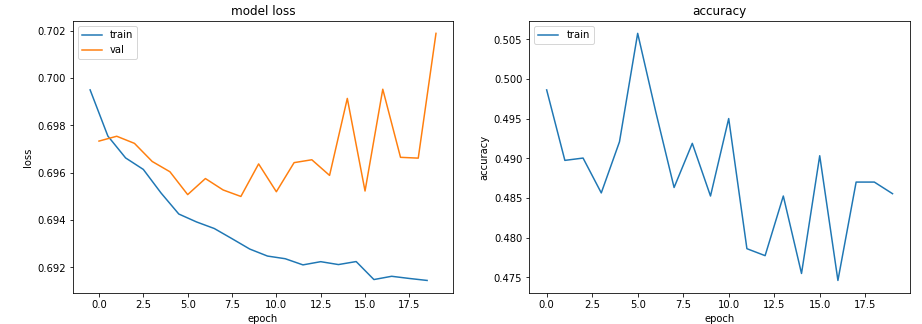Scenario 1 — Model seems to learn, but does not perform well on Validation or Accuracy

## Scenario 1 — Possible Reason — ‘Not enough information in the data to allow “learning”’

It is possible that the training data does not contain sufficient information to allow the model to ‘learn’.

In this case (in the code I am assigning the train data to be just random data) it means it can’t learn anything of substance.

It is always imperative that the data has sufficient information to be able to learn from. Remember, EDA and Feature Engineering are key !! Models learn what can be learnt. Not make up what doesn’t exist.

# Scenario 2 — Train, Val and Accuracy curves all really choppy and not settling

• Learning Rate = 0.1
• Batch Size = 128 (default)
`class Scenario_2_Model_1(nn.Module):    def __init__(self, in_features=30, out_features=2):        super().__init__()        self.lin1 = nn.Linear(in_features, out_features)    def forward(self, x):        x = self.lin1(x)        return xget_data_train_and_show(Scenario_2_Model_1(), lr=0.1)`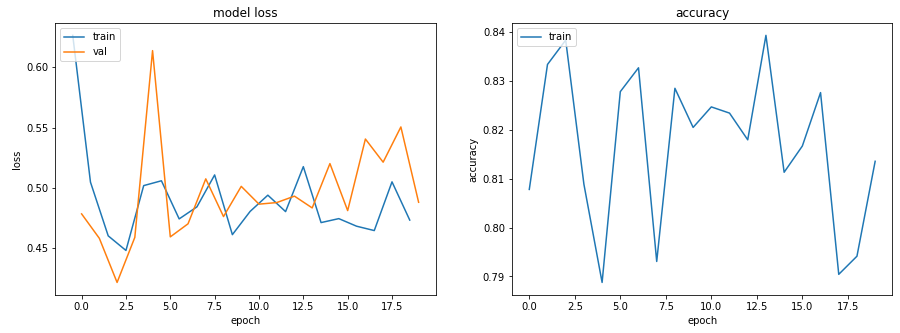Scenario 2 — Model doesn't seem to want to learn — all graphs choppy

## Scenario 2 — Possible Reason — ‘Learning rate is too high’ or ‘batch size too low’

Reduce the learning rate from 0.1 to 0.001, means it won’t ‘bounce around’ and will instead smoothly reduce.

`get_data_train_and_show(Scenario_1_Model_1(), lr=0.001)`

As well as reducing the learning rate, increasing the batch size will also make it smoother.

`get_data_train_and_show(Scenario_1_Model_1(), lr=0.001, batch_size=256)`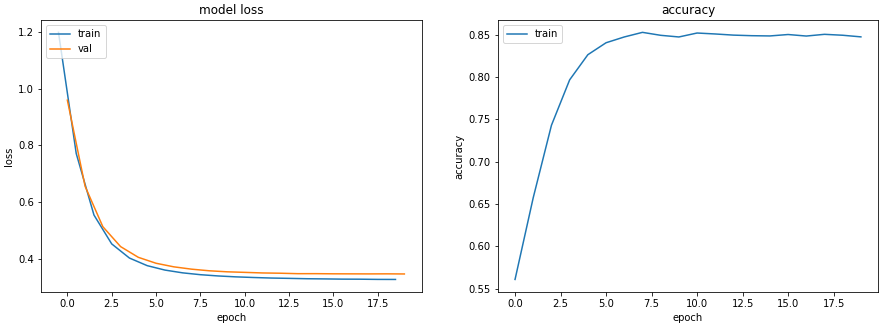Scenario 2 —With a Reduced Learning Rate and Increased Batch Size

# Scenario 3 — Train Loss goes to nearly zero and the Accuracy is looking OK, but the Val doesn’t drop as you would like, and then it just starts to rise

`class Scenario_3_Model_1(nn.Module):    def __init__(self, in_features=30, out_features=2):        super().__init__()        self.lin1 = nn.Linear(in_features, 50)        self.lin2 = nn.Linear(50, 150)        self.lin3 = nn.Linear(150, 50)        self.lin4 = nn.Linear(50, out_features)    def forward(self, x):        x = F.relu(self.lin1(x))        x = F.relu(self.lin2(x))        x = F.relu(self.lin3(x))        x = self.lin4(x)        return xget_data_train_and_show(Scenario_3_Model_1(), lr=0.001)`

## Scenario 3 — Possible Reason — ‘It’s overfitting’

The extremely low training loss and the high accuracy, while the validation loss and training loss are getting wider and wider apart, are all classic overfitting indicators.

Fundamentally, your model has too much capacity to learn. It is memorizing the training data too well, but that of course means it can’t generalize to new data as well. (Note that the model we used (Scenario_3_Model_1) has several layers and quite a high number of parameters)

First thing we could try is reduce the complexity of the model.

`class Scenario_3_Model_2(nn.Module):    def __init__(self, in_features=30, out_features=2):        super().__init__()        self.lin1 = nn.Linear(in_features, 50)        self.lin2 = nn.Linear(50, out_features)    def forward(self, x):        x = F.relu(self.lin1(x))        x = self.lin2(x)        return xget_data_train_and_show(Scenario_3_Model_2(), lr=0.001)`

That made it a lot better, but we can introduce a little L2 Weight Decay regularization to make it even better again (suitable for shallower models).

`get_data_train_and_show(Scenario_3_Model_2(), lr=0.001, wd=0.02)`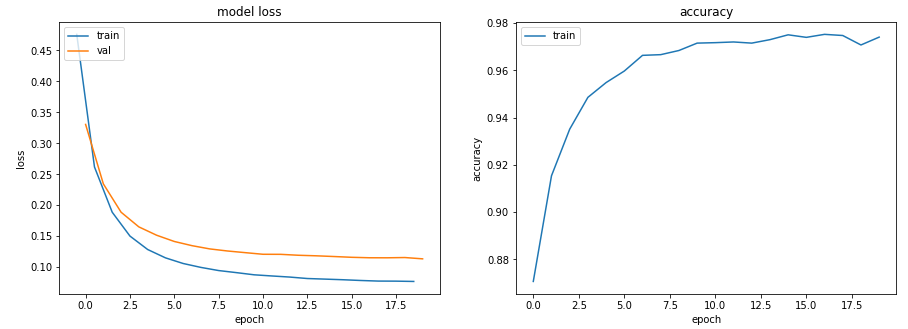Scenario 3 — With reduced model complexity and an L2 Weight Decay

But we could also have kept the model as deep and large as it was, but introduce dropout (suitable for deeper models).

`class Scenario_3_Model_3(nn.Module):    def __init__(self, in_features=30, out_features=2):        super().__init__()        self.lin1 = nn.Linear(in_features, 50)        self.lin2 = nn.Linear(50, 150)        self.lin3 = nn.Linear(150, 50)        self.lin4 = nn.Linear(50, out_features)        self.drops = nn.Dropout(0.4)    def forward(self, x):        x = F.relu(self.lin1(x))        x = self.drops(x)        x = F.relu(self.lin2(x))        x = self.drops(x)        x = F.relu(self.lin3(x))        x = self.drops(x)        x = self.lin4(x)        return xget_data_train_and_show(Scenario_3_Model_3(), lr=0.001)`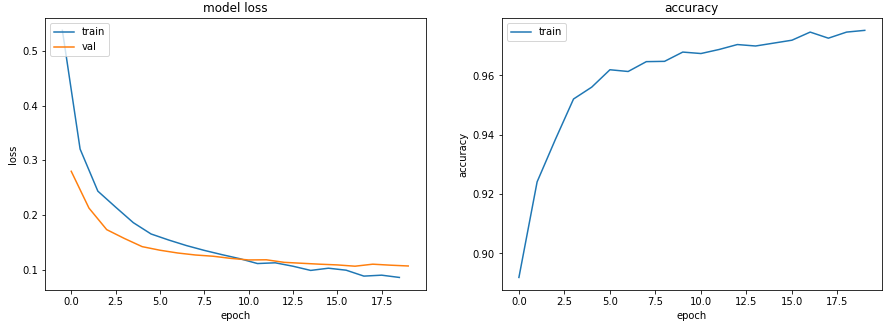Scenario 3 — Still using a deep model, but now with Dropout

# Scenario 4 — Train and Val behaving, but Accuracy just not getting high

• Learning Rate = 0.001
• Batch Size = 128 (default)
• Number of possible classes = 5
`class Scenario_4_Model_1(nn.Module):    def __init__(self, in_features=30, out_features=2):        super().__init__()        self.lin1 = nn.Linear(in_features, 2)        self.lin2 = nn.Linear(2, out_features)    def forward(self, x):        x = F.relu(self.lin1(x))        x = self.lin2(x)        return xget_data_train_and_show(Scenario_4_Model_1(out_features=5), lr=0.001, n_classes=5)`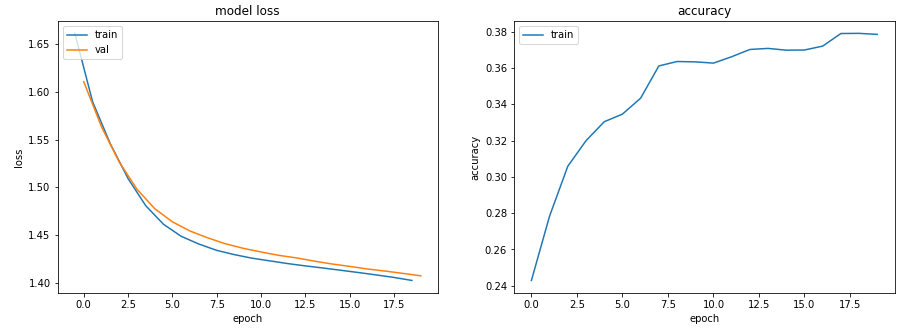Scenario 4 — Train and Val behaving, but Accuracy just not getting higher than 38%

## Scenario 4 — Possible Reason — ‘Not enough capacity to learn’

One of the layers in your model has fewer parameters than there are classes in the possible output of the model. In this case, a layer of 2 in the middle when there are 5 possible output classes.

This means the model is losing some of the individual data about those classes as it is having to cram it through a smaller layer, and so struggles to get that information back once the layers get bigger again.

So make sure the layers never go smaller than the output size of the model.

`class Scenario_4_Model_2(nn.Module):    def __init__(self, in_features=30, out_features=2):        super().__init__()        self.lin1 = nn.Linear(in_features, 50)        self.lin2 = nn.Linear(50, out_features)    def forward(self, x):        x = F.relu(self.lin1(x))        x = self.lin2(x)        return xget_data_train_and_show(Scenario_4_Model_2(out_features=5), lr=0.001, n_classes=5)`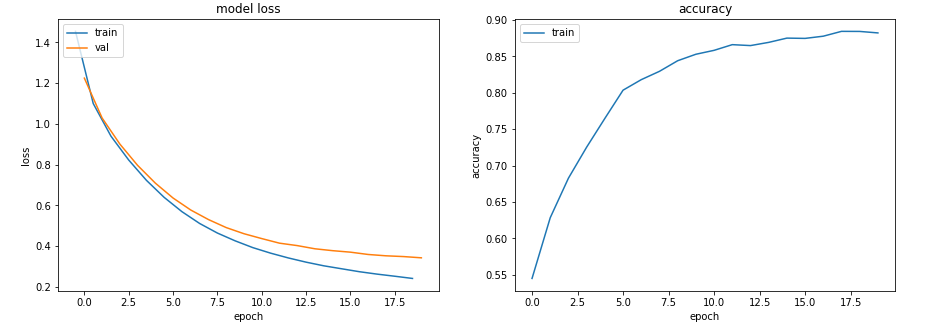Scenario 4 — Ensuring the layers never go smaller than the number of classes, and accuracy up at 88%

# Conclusion

And there you have it. Examples of different Training, Validation and Accuracy graphs, what they are telling you and how you can make them better.

I hope this has been helpful.

--

--

--

## More from Martin Keywood

Data Science and Machine Learning are hugely exciting, enabling better data understanding to see beyond the obvious and help make decisions and help patients.

Love podcasts or audiobooks? Learn on the go with our new app.

## Vid2Vid — Conditional GANs for Video-to-Video Synthesis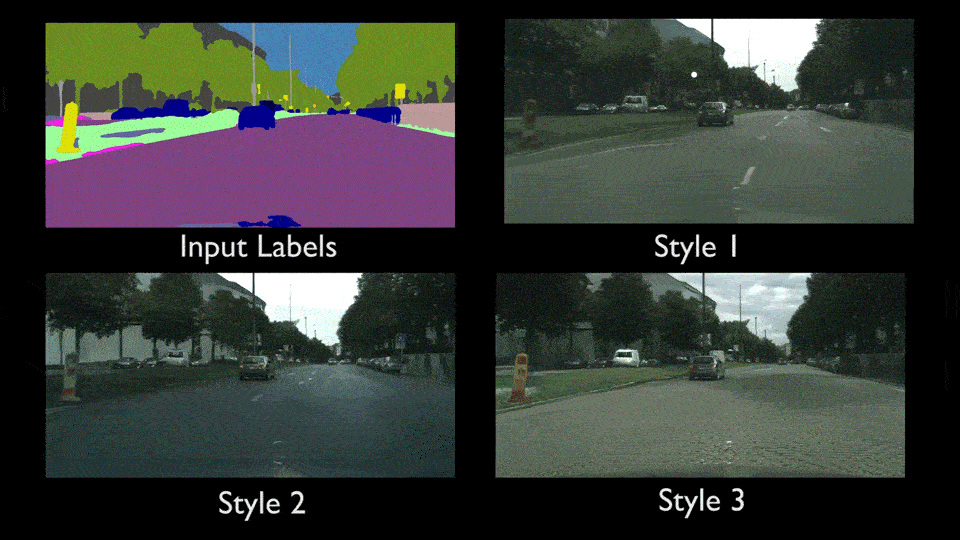## Try Tensorflow Line by Line Tutorial Part 1## Audio Source Separation with Deep Learning## Further reduce your time to AI with deep learning templates## Logistic Regression: The baseline classification model Explained## Making data meaningless so AI can map its meaning## (PPS) Dynamic Routing Between Capsules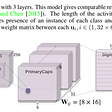## Revealing what neural networks see and learn: PytorchRevelio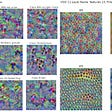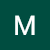## Martin Keywood

Data Science and Machine Learning are hugely exciting, enabling better data understanding to see beyond the obvious and help make decisions and help patients.

## ML model evaluation using Cross Validation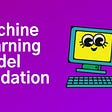## Top Activation Functions at a glance## Explore and Validate Datasets with TensorFlow Extended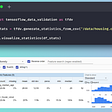## Pytorch Vs Keras Deep Neural Network for Mobile Price Prediction.